StatLect

# Domain shift

Domain shift (or distributional shift) is a major problem that may negatively affect the performance of our machine learning models when we put them in production.

Domain shift happens when our training, validation and test data are drawn from a probability distribution that is different from the distribution of the data on which we will use our predictive models.

Several problems that are well-known in machine learning and statistics (e.g., dependence structures, randomization bias, structural breaks, non-representative samples) can be seen as special cases of domain shift.

One of the main consequences of domain shift is that our estimates of the expected loss on the test set may be biased.

While domain shift is hard to overcome, we can gauge its adverse effects on out-of-sample predictions by taking special precautions when we form our test samples.## Theory

Denote by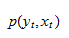the joint probability distribution of outputsand inputs, from whichandwill be extracted when our predictive models are put in production.

Domain shift happens when the data used for training, validation and testing is not drawn from, but it is instead drawn from the conditional distributionwhereis some random variable that we may not observe,is not independent fromand, andis a proper subset of the support of.

In other words, domain shift happens when the data used for model building is generated under particular conditions (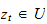) that will not necessarily continue to hold when our predictive models are put in production.

Note that, on the one hand, the conditioning informationcan be seen as inducing dependence among the observations. On the other hand, the lack of independence among observations can be seen as a particular case of domain shift (think ofas a stochastic process on whichandare dependent and that explores only a limited portionof its state-space while we gather our data).

## Examples

Let us make two examples.

### Time-series data

Suppose thatdenotes time, that is, we are dealing with time-series data.

If our data describes some economic or financial phenomenon, there may be background conditions (e.g., economic policies, market microstructure, demographics) that held during the period in which our data was collected, but that may not necessarily hold in the future. In this case, the information on which we are conditioning () is a specific set of background conditions.

### Randomization bias

Suppose that our observations pertain to a sample of individuals drawn from a population.

If those individuals are scarcely representative of the population, then we will encounter a different distribution of the data when we use our predictive models for new individuals drawn at random from the population. In this case, the information on which we are conditioning () is a particular segment of the population.

## Consequences for testing

Let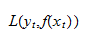be the loss incurred when we predict the outputwith a predictive model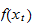.

Suppose that we have drawn the test set at random from our data set and that domain shift afflicts our prediction problem.

Then, the empirical risk (average loss) of a predictive modelon the test set is an unbiased estimate of the conditional expectation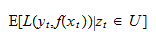However, it may be a biased estimate of the expectation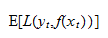which is the actual expected loss that we will face when we put our model in production.

## Blocking

Blocking is a heuristic technique that allows us to gauge the possible extent of the bias in our estimates of the expected loss. It allow us to use the test set to understand what may happen when our predictive models face data coming from a different distribution.

The main idea is to try and split our data in such a way that the distribution of the data in the training and validation samples is different from the distribution in the test sample, so as to mimic what happens when we put the predictive models in production. In other words, we simulate what happens to the expected loss when the models have been trained and validated using the wrong distribution.

Note that this method neither solves the domain-shift problem nor provides an unbiased estimate of the expected loss. However, it provides a tentative quantification of the adverse effects of domain shift.

## Examples of blocking strategies

Let us continue with the two examples above.

### Time-series data

If we suspect that our time-series data may be affected by domain shift, we can perform blocking by putting blocks of data that are contiguous in time in our test sample.

For example, if our sample covers the period 1980-2020, we can use all the data pertaining to the period 2012-2020 for testing purposes (and the sample 1980-2011 for training and validation). In this manner, we are able to assess the ability of our models to make predictions during previously unseen time spans.

If we are performing K-fold cross-validation, then we need to put time-contiguous data in each fold. In this manner, we separate data that may come from different distributions (because of structural breaks / changes in background conditions that happen through time).

### Randomization bias

Suppose that our sample is made by repeated observations made on some individuals.

For example, we observe the amounts spent by the customers of an online shop (output) during one year, together with some potentially helpful predictors (inputs). Each customer is identified by a unique ID and some customers return more than once to the online shop.

The problem of predicting amounts spent is prone to domain shift because the customers coming to the shop in the future may be systematically different from those that came during the data-collection year (e.g., because we launch a new advertising campaign).

The first thing we can do is blocking at the individual level, that is, we put all the observations pertaining to some individuals in the test sample, so that no observation pertaining to those same individuals is in the training or validation samples. In this manner, testing allows us to gauge the ability of our models to make predictions for previously unseen individuals.

We can also perform blocking at the group level, based on variables that allow us to assign our individuals to different segments. For example, if we previously run several different advertisement campaigns, we can segment the customers based on the campaign that referred them to our online shop. Then, we put some segments only in the test set and the remaining segments only in the training and validation set. By doing so, testing allows us to assess the ability of our models to make predictions for previously unseen segments (a new advertising campaign).

## K-fold cross-validation

It is probably needless to say, but, when doing blocking, it is especially advantageous to perform K-fold cross validation, which allows us to simulate the performance of our models across many potentially different distributional shifts.

We have seen how to better estimate the expected loss of our models in the presence of domain shift. But what about training models that are robust to domain shift from the outset? Can we build features that are good predictors irrespective of the subset ofon which we are conditioning? This is a very young field that is open for research. Try and run a Google search for domain adaptation or have a look at the relevant Wikipedia article.

## Bottom line

The main take-away is: if you suspect that something may go wrong when you put your predictive models into production, try to simulate that unfortunate something by constructing your test set in a smart way.

## Python example: inflation data set

We have previously dealt with the inflation data set, although ignoring the fact that inflation data, being time-series data, may be affected by domain shift. We now see what happens when we use blocking to test a boosted linear regression model used to predict inflation.

### Import the data

We first import the data.

# Import the packages used to load and manipulate the data
import numpy as np # Numpy is a Matlab-like package for array manipulation and linear algebra
import pandas as pd # Pandas is a data-analysis and table-manipulation tool

# Import the function that performs sample splits from scikit-learn
from sklearn.model_selection import train_test_split

try:
except:
y = y.values # Transform y into a numpy array

# Print some information about the output variable
print('Class and dimension of output variable:')
print(type(y))
print(y.shape)

# Load the input variables with pandas
try:
except:
x = x.values

# Print some information about the input variables
print('Class and dimension of input variables:')
print(type(x))
print(x.shape)

The output is:

Class and dimension of output variable:
class 'numpy.ndarray'
(270, 1)
Class and dimension of input variables:
class 'numpy.ndarray'
(270, 113)

### Create a boosted linear regression class

We create our own class for training boosted linear regression models.

# Import package used to make copies of objects
from copy import deepcopy

# Our boosted linear regression (blr) class will implement 3 methods
# (constructor, fit, and predict), as previously seen in scikit-learn
class blr:
def __init__(self, learning_rate, max_iter, early_stopping):
self.lr = learning_rate
self.max_iter = max_iter
self.early = early_stopping
self.y_mean = 0
self.y_std = 1
self.x_mean = 0
self.x_std = 1
self.theta = 0
self.mses = []

def fit(self, x_train_0, y_train_0, x_val_0, y_val_0):
# Make copies of data to avoid over-writing original dataset
x_train = deepcopy(x_train_0)
y_train = deepcopy(y_train_0)
x_val = deepcopy(x_val_0)
y_val = deepcopy(y_val_0)

# De-mean the output variable
self.y_mean = np.mean(y_train)
y_train -= self.y_mean
y_val -= self.y_mean

# Standardize the output variable
self.y_std = np.std(y_train)
y_train /= self.y_std
y_val /= self.y_std

# De-mean the input variables
self.x_mean = np.mean(x_train, axis=0, keepdims=True)
x_train -= self.x_mean
x_val -= self.x_mean

# Standardize the input variables
self.x_std = np.std(x_train, axis=0, keepdims=True)
x_train /= self.x_std
x_val /= self.x_std

# Initialize counters (total boosting iterations and unproductive iterations)
current_iter = 0
no_improvement = 0

# The starting model has all coefficients equal to zero and predicts a constant zero output
self.theta = np.zeros((x_train.shape, 1))
y_train_pred = 0 * y_train
y_val_pred = 0 * y_val
eta = y_train - y_train_pred
mses = [np.var(y_val - y_val_pred)]

# Boosting iterations
while no_improvement < self.early and current_iter < self.max_iter:
current_iter += 1
corr_coeffs = np.mean(x_train * eta, axis=0) # Correlations (equal to betas) beteen residual and inputs
index_best = np.argmax(np.abs(corr_coeffs)) # Choose variable that has maximum correlation with residual
self.theta[index_best] += self.lr * corr_coeffs[index_best] # Parameter update
y_train_pred += self.lr * corr_coeffs[index_best] * x_train[:, [index_best]] # Prediction update
eta = y_train - y_train_pred # Residuals update
y_val_pred += self.lr * corr_coeffs[index_best] * x_val[:, [index_best]] # Validation prediction update
mses.append(np.var(y_val - y_val_pred)) # New validation MSE
if mses[-1] > np.min(mses[0:-1]): # Stopping criterion to avoid over-fitting
no_improvement += 1
else:
no_improvement = 0

def predict(self, x_test_0):
# Make copies of the data to avoid over-writing original dataset
x_test = deepcopy(x_test_0)

# De-mean input variables using means on training sample
x_test = x_test - self.x_mean

# Standardize output variables using standard deviations on training sample
x_test = x_test / self.x_std

# Return prediction
return self.y_mean + self.y_std * np.dot(x_test,self.theta)

### Standard K-fold cross-validation of the boosted linear regression model

We first run a standard K-fold cross-validation of the boosted linear regression model.

# Import the functions that performs sample splits from scikit-learn
from sklearn.model_selection import train_test_split, KFold

# Import model-evaluation metrics from scikit-learn
from sklearn.metrics import mean_squared_error, r2_score

# Set number of folds and ensemble variables
n_folds = 5
ensemble = []
mses_single_models = []
mses_constant_predictions = []
r_squareds_single_models = []

# Initialize k_fold splitter
K_fold = KFold(n_splits=n_folds, random_state=0, shuffle=True)

# Iterate over folds
for train_val_index, test_index in K_fold.split(x):
# Get train_val (K-1 folds) and test (1 fold)
x_train_val, x_test = x[train_val_index], x[test_index]
y_train_val, y_test = y[train_val_index], y[test_index]

# Partition the train_val set
x_train, x_val, y_train, y_val
= train_test_split(x_train_val, y_train_val, test_size=0.25, random_state=0)

# Create a boosted linear regression object
lr = blr(0.1, 10000, 20)

# Train the model
lr.fit(x_train, y_train, x_val, y_val)

# Save the model in the ensemble list
ensemble.append(lr)

# Make predictions on test and compute performance metrics
y_test_pred = lr.predict(x_test)
mses_single_models.append(mean_squared_error(y_test, y_test_pred))
mses_constant_predictions.append(mean_squared_error(y_test, 0*y_test + np.mean(y_train)))
r_squareds_single_models.append(r2_score(y_test, y_test_pred))

# Print performance metrics on test sample
print('Test MSEs of models in the ensemble:')
print(mses_single_models)
print('Test MSEs of constant predictions equal to sample mean on training set:')
print(mses_constant_predictions)
print('Average test MSE of models in the ensemble:')
print(np.mean(mses_single_models))
print('')

print('Test R squareds of models in the ensemble:')
print(r_squareds_single_models)
print('Average test R squared of models in the ensemble:')
print(np.mean(r_squareds_single_models))


The output is:

Test MSEs of models in the ensemble:
[0.0822823793036502, 0.0713880523366307, 0.07973393791858635, 0.0586962842821171, 0.06410835284278463]
Test MSEs of constant predictions equal to sample mean on training set:
[0.24976934245574184, 0.14997980939756583, 0.19739643607446342, 0.14594386864523798, 0.1760051803216836]
Average test MSE of models in the ensemble:
0.0712418013367538

Test R squareds of models in the ensemble:
[0.6657386162590521, 0.523397645936722, 0.5876162214584426, 0.5977449324774733, 0.6357482079607049]
Average test R squared of models in the ensemble:
0.6020491248184789


### Blocked K-fold cross-validation of the boosted linear regression model

In order to perform blocking, we change a single line of code: we set the shuffle option to False in the scikit-learn K-fold splitter. The code contains a commented line that allows to print the blocks. Uncomment it if you want to check how blocking has been performed.

# Import the functions that performs sample splits from scikit-learn
from sklearn.model_selection import train_test_split, KFold

# Import model-evaluation metrics from scikit-learn
from sklearn.metrics import mean_squared_error, r2_score

# Set number of folds and ensemble variables
n_folds = 5
ensemble = []
mses_single_models = []
mses_constant_predictions = []
r_squareds_single_models = []

# Initialize k_fold splitter
K_fold = KFold(n_splits=n_folds, shuffle=False) # shuffle=False puts adjacent data into folds (blocking)

# Iterate over folds
for train_val_index, test_index in K_fold.split(x):
# print(test_index) # Uncomment this line to print the blocks

# Get train_val (K-1 folds) and test (1 fold)
x_train_val, x_test = x[train_val_index], x[test_index]
y_train_val, y_test = y[train_val_index], y[test_index]

# Partition the train_val set
x_train, x_val, y_train, y_val
= train_test_split(x_train_val, y_train_val, test_size=0.25, random_state=0)

# Create a boosted linear regression object
lr = blr(0.1, 10000, 20)

# Train the model
lr.fit(x_train, y_train, x_val, y_val)

# Save the model in the ensemble list
ensemble.append(lr)

# Make predictions on test and compute performance metrics
y_test_pred = lr.predict(x_test)
mses_single_models.append(mean_squared_error(y_test, y_test_pred))
mses_constant_predictions.append(mean_squared_error(y_test, 0*y_test + np.mean(y_train)))
r_squareds_single_models.append(r2_score(y_test, y_test_pred))

# Print performance metrics on test sample
print('Test MSEs of models in the ensemble:')
print(mses_single_models)
print('Test MSEs of constant predictions equal to sample mean on training set:')
print(mses_constant_predictions)
print('Average test MSE of models in the ensemble:')
print(np.mean(mses_single_models))
print('')

print('Test R squareds of models in the ensemble:')
print(r_squareds_single_models)
print('Average test R squared of models in the ensemble:')
print(np.mean(r_squareds_single_models))

The output is:

Test MSEs of models in the ensemble:
[0.0761299830943787, 0.0314655164015944, 0.07812543324243054, 0.14245825623750002, 0.07018648607198136]
Test MSEs of constant predictions equal to sample mean on training set:
[0.04825032951254399, 0.09639427404386022, 0.231980776581077, 0.3252981875540617, 0.2198194916894304]
Average test MSE of models in the ensemble:
0.07967313500957701

Test R squareds of models in the ensemble:
[-0.6745471938950964, 0.6586478082374208, 0.6612767816751719, 0.5501760861785623, 0.6800297153350183]
Average test R squared of models in the ensemble:
0.3751166395062154

It was a good idea to perform blocking! The estimated performance degrades significantly, although on a single block (the first one). There is probably a structural break at the very beginning of the sample. Nevertheless, the model performs very well on the other parts of the sample.

## References

Chen, Y., Wei, C., Kumar, A. and Ma, T., 2020. Self-training avoids using spurious features under domain shift. arXiv preprint arXiv:2006.10032.

Roberts, D.R., Bahn, V., Ciuti, S., Boyce, M.S., Elith, J., Guillera Arroita, G., Hauenstein, S., Lahoz, and Monfort, J.J., Schroeder, B., Thuiller, W. and Warton, D.I., 2017. Cross-validation strategies for data with temporal, spatial, hierarchical, or phylogenetic structure. Ecography, 40(8), pp.913-929.